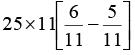Courses

# Quant Mock Test - 14

## 15 Questions MCQ Test Mock Test Series for CLAT 2020 | Quant Mock Test - 14

Description
This mock test of Quant Mock Test - 14 for CLAT helps you for every CLAT entrance exam. This contains 15 Multiple Choice Questions for CLAT Quant Mock Test - 14 (mcq) to study with solutions a complete question bank. The solved questions answers in this Quant Mock Test - 14 quiz give you a good mix of easy questions and tough questions. CLAT students definitely take this Quant Mock Test - 14 exercise for a better result in the exam. You can find other Quant Mock Test - 14 extra questions, long questions & short questions for CLAT on EduRev as well by searching above.
QUESTION: 1

### Directions: Study the following graph and answer the questions given below: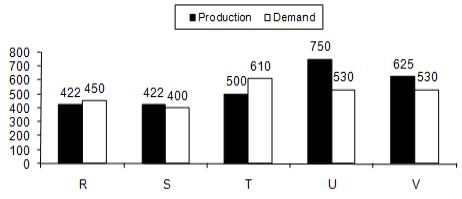Q. The demand of refrigerator of company ‘S’ was approximately what percent of company ‘R’?

Solution:

Required percentage
= 400 / 450 x 100 = 800 / 9%

QUESTION: 2

### Directions: Study the following graph and answer the questions given below:Q. The difference between the average production and the average demand of the five companies taken together is?

Solution:

Avg production of the five companies = 2719/5 = 543.8
Avg demand of the five companies = 2520/5 = 504
Difference = 543.8 – 504 = 39.8

QUESTION: 3

### Directions: Study the following graph and answer the questions given below:Q. Which two companies have equal demand for refrigerators?

Solution:

U and V have equal demands for refrigerator, each = 530.

QUESTION: 4

Directions: Study the following graph and answer the questions given below:Q. Which of the following companies has the largest difference between demand and supply?

Solution:

U has the largest difference between production and demand = 750 – 530 = 220

QUESTION: 5

Directions: Study the following graph and answer the questions given below:Q. Which companies have their productions greater than the average of all the five companies taken together?

Solution:

Avg production of the five companies = 2719 / 5 = 543.8
In two years production is more than average production.

QUESTION: 6

Directions: In the following line graph the goods produced by a company (in tonnes) from 2001 to 2008 is shown. On this basis answer the following questions.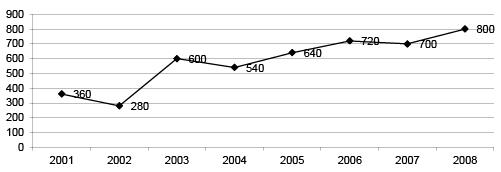Q. The average amount of production from 2001 to 2005 is

Solution: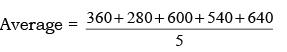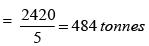QUESTION: 7

Directions: In the following line graph the goods produced by a company (in tonnes) from 2001 to 2008 is shown. On this basis answer the following questions.Q. The ratio of maximum to minimum quantity produced during 2001-08 is

Solution: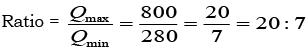QUESTION: 8

Directions: In the following line graph the goods produced by a company (in tonnes) from 2001 to 2008 is shown. On this basis answer the following questions.Q. The maximum percentage rise in production from its previous years is

Solution:

It is in 2003, % increase=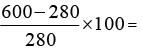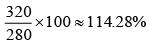QUESTION: 9

Directions: In the following line graph the goods produced by a company (in tonnes) from 2001 to 2008 is shown. On this basis answer the following questions.Q. The percentage rise in production from 2003 to 2008 is

Solution:

In 2003, it is 600. In 2008, it is 800.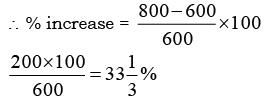QUESTION: 10

Directions: In the following line graph the goods produced by a company (in tonnes) from 2001 to 2008 is shown. On this basis answer the following questions.Q. What is the total production from 2001 to 2008?

Solution:

Total production = 360+280+600+540+640+720+700+800 =  4640 tonnes.

QUESTION: 11

Directions: Study the following information carefully to answer these questions.
Percentage of employees in various departments of an organization and the male female ratio Total no. of Employees = 2500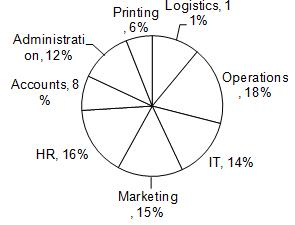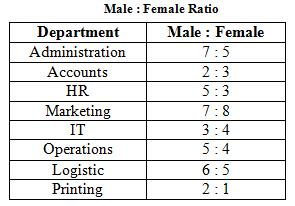Q. What is the ratio of male employees in Administration to those in Printing Department?

Solution:

Ratio = 12 x 7/12 : 6 x 2/3 = 7 : 4

QUESTION: 12

Directions: Study the following information carefully to answer these questions.
Percentage of employees in various departments of an organization and the male female ratio Total no. of Employees = 2500Q. What is the difference between the total number of employees in IT and that in Operations Department?

Solution:

4% of 2500 = 100

QUESTION: 13

Directions: Study the following information carefully to answer these questions.
Percentage of employees in various departments of an organization and the male female ratio Total no. of Employees = 2500Q. What is the ratio of the total number of males in HR and Marketing to the total number of females in these two departments?

Solution:

Ratio = (16 x 5/8 + 15 x 7/15) : (16 x 3/8 + 15 x 8/15) = 17 : 14

QUESTION: 14

Directions: Study the following information carefully to answer these questions.
Percentage of employees in various departments of an organization and the male female ratio Total no. of Employees = 2500Q. How many female employees are there in the HR Department?

Solution:

3/8 x 16 x 25 = 150

QUESTION: 15

Directions: Study the following information carefully to answer these questions.
Percentage of employees in various departments of an organization and the male female ratio Total no. of Employees = 2500Q. What is the difference between the numbers of male and female employees in Logistics Department?

Solution: